Courses

# Determinacy and Indeterminacy Civil Engineering (CE) Notes | EduRev

## Civil Engineering (CE) : Determinacy and Indeterminacy Civil Engineering (CE) Notes | EduRev

The document Determinacy and Indeterminacy Civil Engineering (CE) Notes | EduRev is a part of the Civil Engineering (CE) Course Topic wise GATE Past Year Papers for Civil Engineering.
All you need of Civil Engineering (CE) at this link: Civil Engineering (CE)

Q.1 The degree of static indeterminacy of the plane frame as shown in the figure is______    [2019 : 1 Mark, Set-II]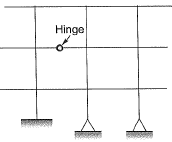Solution:

Dse = 7-3 = 4
Dsi = (3 x 4)-(2--1)= 11
Ds = 15

Q.2  Consider the frame shown in the figure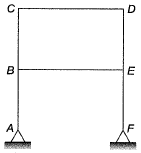If the axial and shear deformations in different members of the frame are assumed to be negligible, the reduction in the degree of kinematic indeterminacy would be equal to    [2017 : 1 Mark, Set-II]
(a) 5
(b) 6
(c) 7
(d) 8
Ans.
(B)
Solution:
DOF of Joints: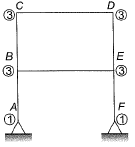When all members are extensible,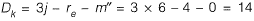Dk (when extensible) = 14
Dk(when inextensible) = Dk (when extensible) - No. of axially rigid member
= 14 - 6 = 8
So, reduction in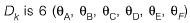Note : Shear deformation is not considered in calculation of DK.

Q.3 A planar truss tower structure is shown in the figure.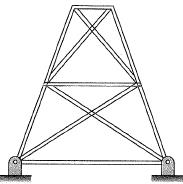Consider the following statements about the external and internal determinacies of the truss.
P. Externally Determinate
Q. External Static indeterminacy = 1
R. External Static Indeterminacy = 2
S. Internally Determinate
T. Internal Static Indeterminacy = 1
U. Internal Static Indeterminacy = 2
Which one of the following options is correct?    [2017 : 2 Marks, Set-I]
(a) P-False; Q-True; R-False; S-False; T-False; U-True
(b) P-False; Q-True; R-False; S-False; T-True; U-False
(c) P-False; Q-False; R-True; S-False; T-False; U-True
(d) P-True; Q-True; R-False; S-True; T-False; U-True
Ans.
(A)
Solution:
Dse= re - 3 - s = 4 - 3 = 1
Dsi=m -(2j - 3) = 15 - (2 x 8 - 3)
= 2
Trick : For a truss formed due to combination of simple triangles.
Dsi = No. of double diagonal panels = 2
Dse = R - 3 = 4 - 3 = 1

Q.4 The kinematic indeterminacy of the plane truss shown in the figure is    [2016 : 1 Mark, Set-II]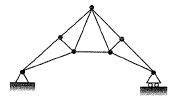(a) 11
(b) 8
(c) 3
(d) 0
Solution:

Kinematic indeterminacy,
D= 2j - re
= 2 x 7 - 3 = 11

Q.5  A guided support as shown in the figure below is represented by three springs (horizontal, vertical and rotational) with stiffness kx, ky and ke respectively. The limiting values of kx, ky and ke are   [2015 : 1 Mark, Set-II]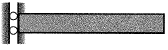(a) ∞, 0, ∞
(b) ∞,∞,∞
(c) 0,∞,∞
(d) ∞,∞,0
Ans.
(A)
Solution:
Guided roller support has 2 reactions: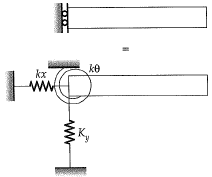For given support, kx = ∞, ky = 0, kθ = ∞

Q.6 The static in determinacy of the two-span continuous beam with an internal hinge, shown below, is ____________ .    [2014 : 1 Mark, Set-II]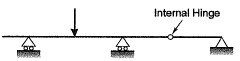Solution:

Number of member,
m = 4
Number of external reaction,
re = 4
Number of joint,
j=5
Number of reaction released,
rr = 1
Degree of static indeterminacy,
Ds = 3 m + re - 3j - rr
= 3 x 4 + 4 - 3 x 5 - 1
= 0

Q.7 The degree of static indeterminacy of a rigid jointed frame PQR supported as shown in figure is    [2014 : 1 Mark, Set-I]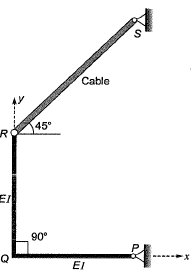(a) zero
(b) one
(c) two
(d) unstable
Ans.
(A)
Solution:
Ds = Dse + Dsi
= (re -3 ) + 3 C - rr,
= (4-3)+ 3 x 0- 1
= 0
Hence, the structure is stable.

Offer running on EduRev: Apply code STAYHOME200 to get INR 200 off on our premium plan EduRev Infinity!

,

,

,

,

,

,

,

,

,

,

,

,

,

,

,

,

,

,

,

,

,

;